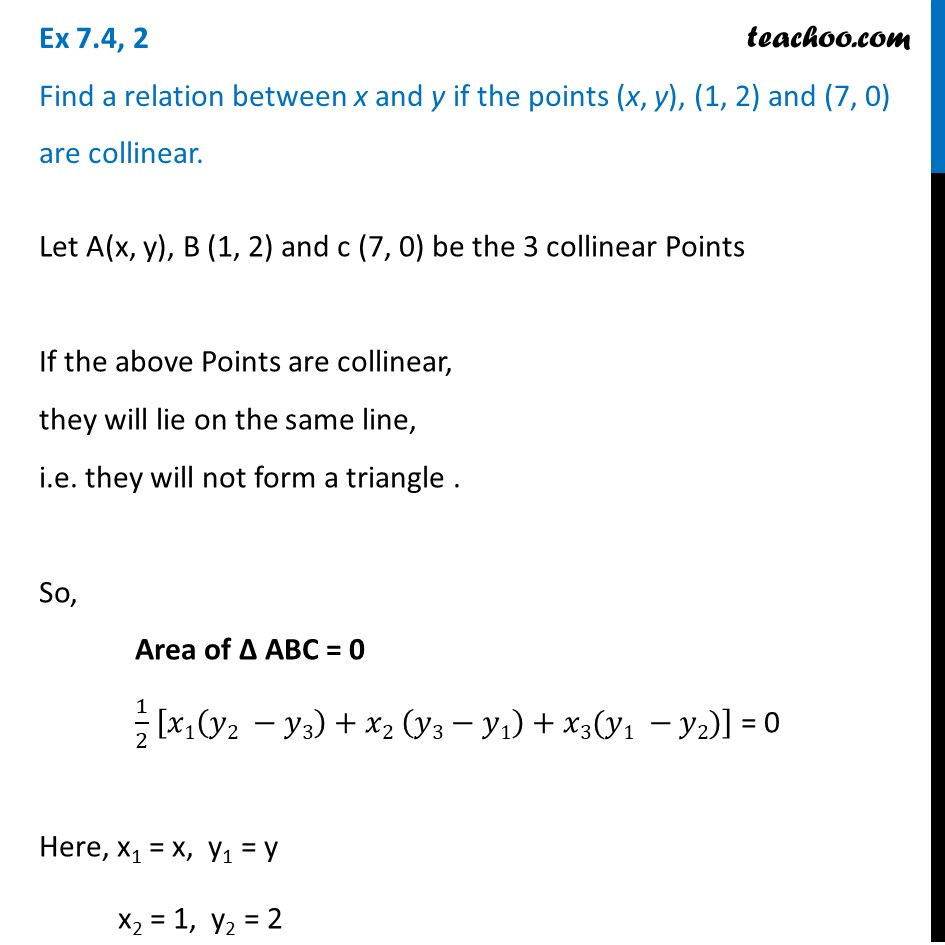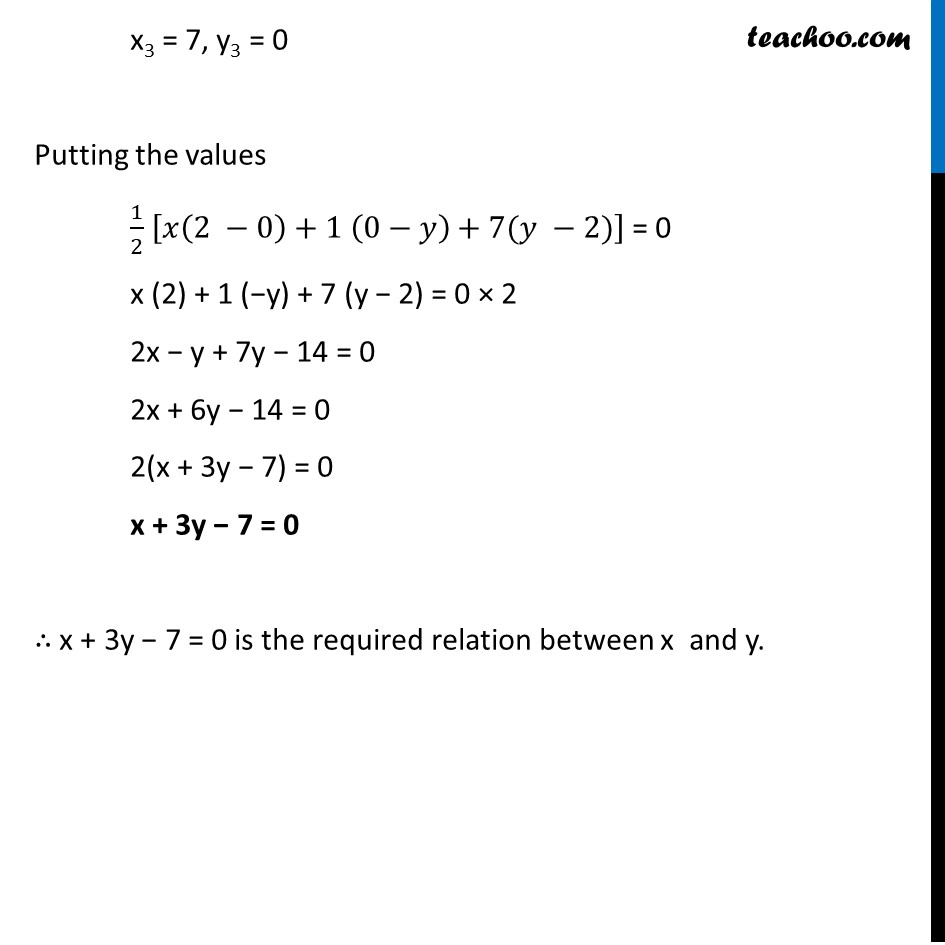Important Coordinate Geometry Questions

Chapter 7 Class 10 Coordinate Geometry
Serial order wiseLearn in your speed, with individual attention - Teachoo Maths 1-on-1 Class

### Transcript

Question 2 Find a relation between x and y if the points (x, y), (1, 2) and (7, 0) are collinear. Let A(x, y), B (1, 2) and c (7, 0) be the 3 collinear Points If the above Points are collinear, they will lie on the same line, i.e. they will not form a triangle . So, Area of ∆ ABC = 0 1/2 [𝑥1(𝑦2 −𝑦3)+𝑥2 (𝑦3−𝑦1)+𝑥3(𝑦1 −𝑦2)] = 0 Here, x1 = x, y1 = y x2 = 1, y2 = 2 x3 = 7, y3 = 0 Putting the values 1/2 [𝑥(2 −0)+1 (0−𝑦)+7(𝑦 −2)] = 0 x (2) + 1 (−y) + 7 (y − 2) = 0 × 2 2x − y + 7y − 14 = 0 2x + 6y − 14 = 0 2(x + 3y − 7) = 0 x + 3y − 7 = 0 ∴ x + 3y − 7 = 0 is the required relation between x and y.